# Measuring Length to the Nearest Centimeter Online Quiz

Following quiz provides Multiple Choice Questions (MCQs) related to Measuring Length to the Nearest Centimeter. You will have to read all the given answers and click over the correct answer. If you are not sure about the answer then you can check the answer using Show Answer button. You can use Next Quiz button to check new set of questions in the quiz.Q 1 - Find the length of the following object to the nearest centimeter.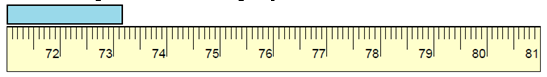### Explanation

Step 1:

The reading is closer to 73 cm than to 74 cm.

Step 2:

So, the length of the given object to the nearest centimeter is 73 cm.

Q 2 - Find the length of the following object to the nearest centimeter.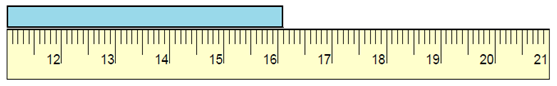### Explanation

Step 1:

The reading is closer to 16 cm than to 17 cm.

Step 2:

So, the length of the given object to the nearest centimeter is 16 cm.

Q 3 - Find the length of the following object to the nearest centimeter.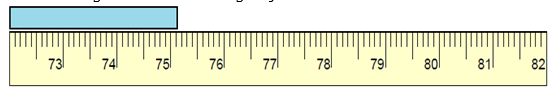### Explanation

Step 1:

The reading is closer to 75 cm than to 76 cm.

Step 2:

So, the length of the given object to the nearest centimeter is 75 cm.

Q 4 - Find the length of the following object to the nearest centimeter.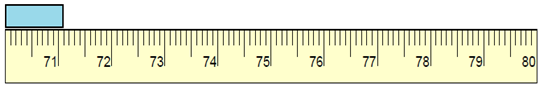### Explanation

Step 1:

The reading is closer to 71 cm than to 72 cm.

Step 2:

So, the length of the given object to the nearest centimeter is 71 cm.

Q 5 - Find the length of the following object to the nearest centimeter.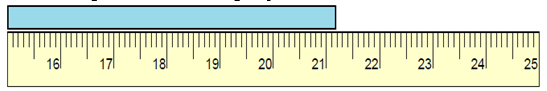### Explanation

Step 1:

The reading is closer to 21 cm than to 22 cm.

Step 2:

So, the length of the given object to the nearest centimeter is 21 cm.

Q 6 - Find the length of the following object to the nearest centimeter.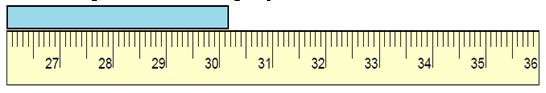### Explanation

Step 1:

The reading is closer to 30 cm than to 31 cm.

Step 2:

So, the length of the given object to the nearest centimeter is 30 cm.

Q 7 - Find the length of the following object to the nearest centimeter.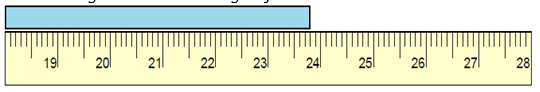### Explanation

Step 1:

The reading is closer to 24 cm than to 23 cm.

Step 2:

So, the length of the given object to the nearest centimeter is 24 cm.

Q 8 - Find the length of the following object to the nearest centimeter.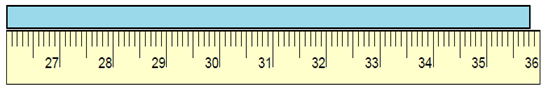### Explanation

Step 1:

The reading is closer to 36 cm than to 35 cm.

Step 2:

So, the length of the given object to the nearest centimeter is 36 cm.

Q 9 - Find the length of the following object to the nearest centimeter.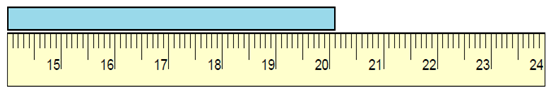### Explanation

Step 1:

The reading is closer to 20 cm than to 21 cm.

Step 2:

So, the length of the given object to the nearest centimeter is 20 cm.

Q 10 - Find the length of the following object to the nearest centimeter.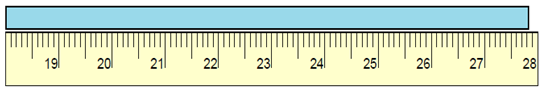### Explanation

Step 1:

The reading is closer to 28 cm than to 27 cm.

Step 2:

So, the length of the given object to the nearest centimeter is 28 cm.

measuring_length_to_the_nearest_centimeter.htm